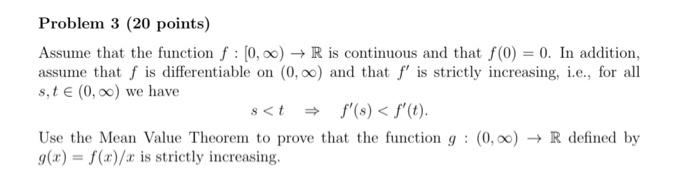# (Solved): Assume that the function f:[0,)R is continuous and that f(0)=0. In addition, assume that f is ...Assume that the function is continuous and that . In addition, assume that is differentiable on and that is strictly increasing, i.e., for all we have Use the Mean Value Theorem to prove that the function defined by is strictly increasing.

We have an Answer from Expert